Select Page

# CBSE Probability Maths 12 Science Answers for MCQ in English

CBSE Probability Maths 12 Science Answers for MCQ in English to enable students to get Answers in a narrative video format for the specific question.

Expert Teacher provides CBSE Probability Maths 12 Science Answers for MCQ through Video Answers in English language. This video solution will be useful for students to understand how to write an answer in exam in order to score more marks. This teacher uses a narrative style for a question from Probability not only to explain the proper method of answering question, but deriving right answer too.

Please find the question below and view the Answer in a narrative video format.

Question:

## Similar Questions from CBSE, 12th Science, Maths, Probability

Question 1 : There are three coins. First is a biased that comes up tails 60% of the times, second is also a biased coin that comes up heads 75% of the times and third is an unbiased coin. One of the three coins is chosen at random and tossed, it shows heads, what is the probability that it was the first coin?      (View Answer Video)

Question 2 :  Four cards are drawn successively with replacement from a well shuffled deck of 52 cards. What is the probability that:

Question 3 : Five bad oranges are accidently mixed with 20 good ones. If four oranges are drawn one by one successively with replacement, then find the probability distribution of number of bad oranges drawn. Hence, find the mean and variance of the distribution.    (View Answer Video)

Question 4 :  A and B throw a pair of dice alternatively, till one of them gets a total of 10 and wins the game. Find their respective probabilities of winning, if A starts first.   (View Answer Video)

### Three Dimensional Geometry

Question 1 : What is the distance of the point (p, q, r) from the x-axis ? (View Answer Video)

Question 2 : Find the equation of the plane passing through the point (-1, 3, 2) and perpendicular to each of the planes x + 2y + 3z = 5 and 3x + 3y + z = 0. (View Answer Video)

Question 3 : Write the vector equation of a line passing through the point (1, -1, 2) and parallel to the line whose equations are,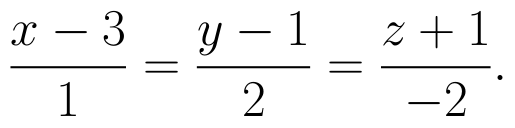(View Answer Video)

Question 4 : Find the co-ordinates of the point where the line through the points A(3, 4, 1) and B(5, 1, 6) crosses the XY-plane. Also find angle which this line makes with the XZ-plane. (View Answer Video)

Question 5 : Write the vector equation of the plane passing through the point (a, b, c) and parallel to the plane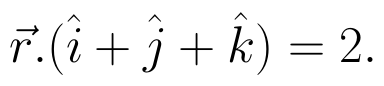(View Answer Video)

### Continuity and Differentiability

Question 1 : If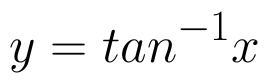, find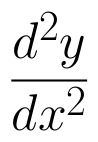in terms of y alone. (View Answer Video)

Question 2 : Findfor the function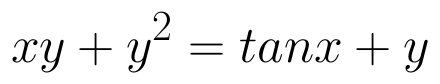. (View Answer Video)

Question 3 : Differentiate the function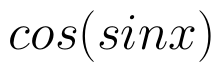with respect to x. (View Answer Video)

Question 4 : Differentiate the function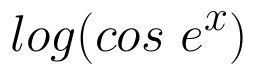with respect to x. (View Answer Video)

Question 5 : Differentiate w.r.t.x the function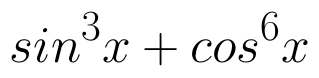. (View Answer Video)

### Application of Integrals

Question 1 : Using the method of integration, find the area of the triangular region whose vertices are (2, -2), (4, 3) and (1, 2). (View Answer Video)

Question 2 :

Find the area of the given curves and given lines: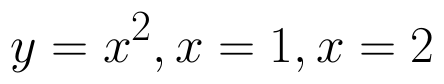and x-axis

Question 3 : Find the area of the region bounded by the curves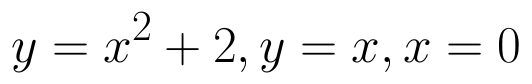and x = 3. (View Answer Video)
Question 4 : Find the area of the region bounded by the parabola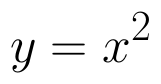and y = | x |. (View Answer Video)
Question 5 : Find the area of the region bounded by the curve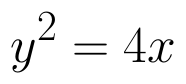and the line x = 3. (View Answer Video)